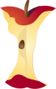Unit Rates and Ratios : The RelationshipPrintable Worksheets And Lessons

• Picnic Step-by-step Lesson- Five bucks for a pizza pie... Can't beat that! How many words can David write in a minute?

• Guided Lesson - This is a regular potpourri of questions for you. We jump from word problems to simple ratios.

• Guided Lesson Explanation - I might have gone a little overboard on the question types here. I have to admit that they are a bit drawn out.

• Practice Worksheet - Word problems for you to walk through. They all assume that proportion hold still.

• Matching Worksheet - This is the most random sheet I have ever created. It just came to me when I was written it.(Click Here to Upgrade)

Homework Sheets

We start with word problems and then move to easily definable proportion problems.

Practice Worksheets

The problems focus on distance and length as the key measurement of focus.

Math Skill Quizzes

The quizzes mimic the other worksheets in this section, but we added some money type problems.

 Quiz 1 Quiz 2 Quiz 3